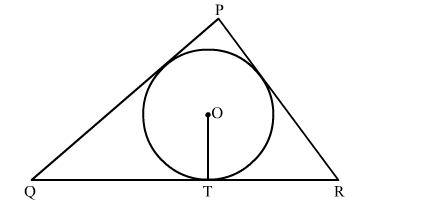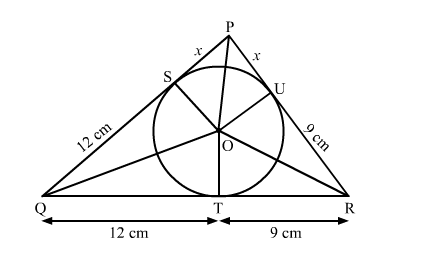# In the given figure, a triangle PQR is drawn to circumscribe a circle of radius 6 cm such that the segments`
Question:

In the given figure, a triangle PQR is drawn to circumscribe a circle of radius 6 cm such that the segments QT and TR into which QR is divided by the point of contact T are of lengths 12 cm and 9 cm respectively. If the area of △PQR = 189 cm2 then the length of side PQ is

(a) 17.5 cm
(b) 20 cm
(c) 22.5 cm
(d) 7.6 cmSolution:We know that tangent segments to a circle from the same external point are congruent.
Therefore, we have
PS = PU = x
QT = QS = 12 cm
RT = RU = 9 cm
Now

$\operatorname{Ar}(\triangle \mathrm{PQR})=\operatorname{Ar}(\triangle \mathrm{POR})+\operatorname{Ar}(\triangle \mathrm{QOR})+\operatorname{Ar}(\triangle \mathrm{POQ})$

$\Rightarrow 189=\frac{1}{2} \times \mathrm{OU} \times \mathrm{PR}+\frac{1}{2} \times \mathrm{OT} \times \mathrm{QR}+\frac{1}{2} \times \mathrm{OS} \times \mathrm{PQ}$

$\Rightarrow 378=6 \times(x+9)+6 \times(21)+6 \times(12+x)$

$\Rightarrow 63=x+9+21+x+12$

$\Rightarrow 2 x=21$

$\Rightarrow x=10.5 \mathrm{~cm}$

Now, PQ = QS + SP = 12 + 10.5 = 22.5 cm
Hence, the correct answer is option (c ).